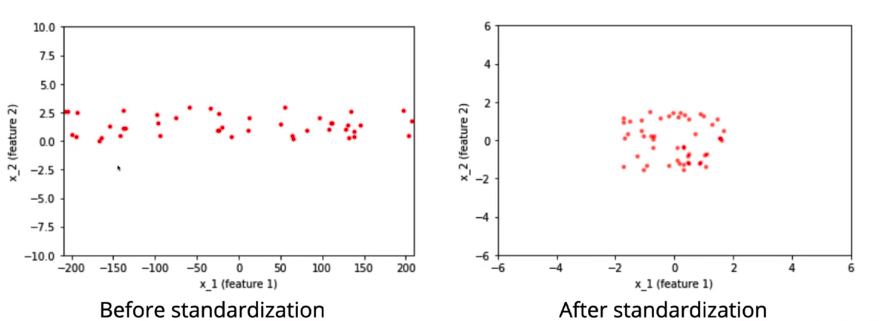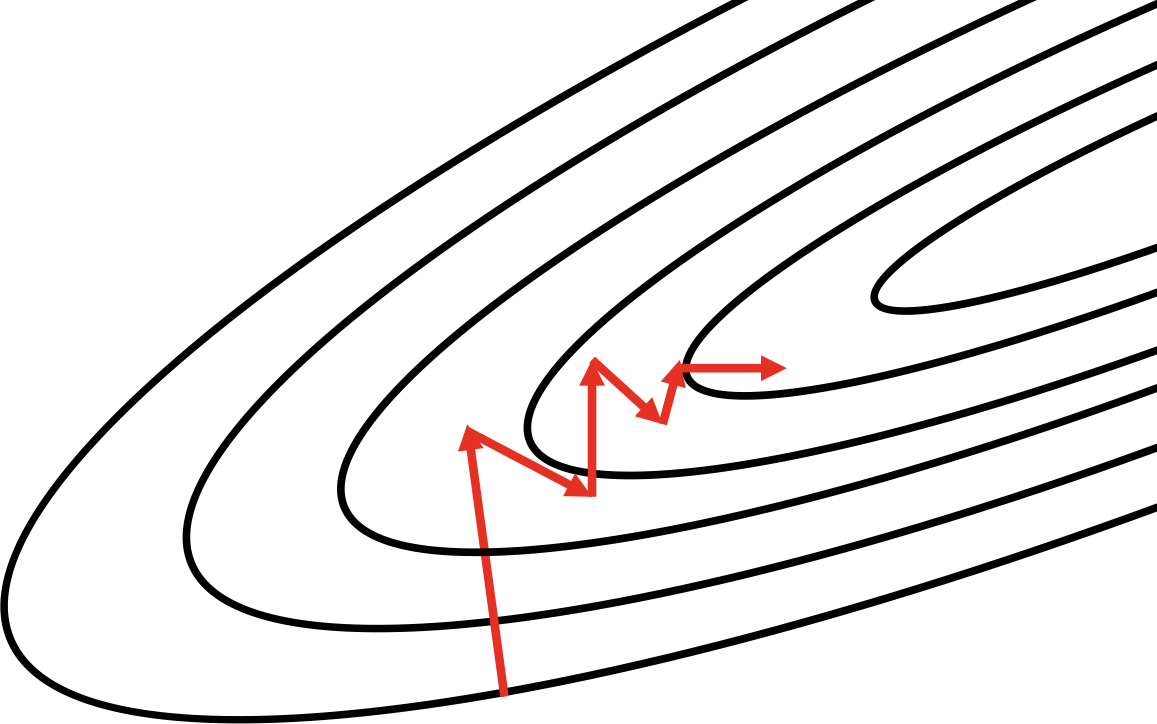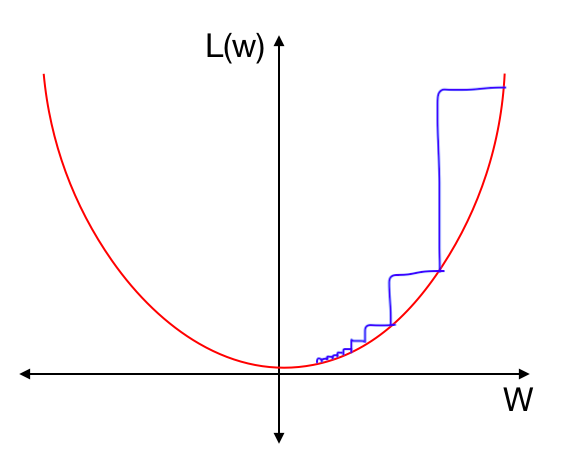1597107300

Batch Normalization, Instance Normalization, Layer Normalization

This short post highlights the structural nuances between popular normalization techniques employed while training deep neural networks.

I am hoping that a quick 2 minute glance at this would refresh my memory on the concept, sometime, in the not so distant future.

Let us establish some notations, that will make the rest of the content, easy to follow. We assume that the activations at any layer would be of the dimensions NxCxHxW (and, of course, in the real number space), where, N = Batch Size, C = Number of Channels (filters) in that layer, H = Height of each activation map, W = Width of each activation map.

#ai #deep-learning #convolutional-network #neural-style-transfer #batch-normalization

Buddha Community1597107300

Batch Normalization, Instance Normalization, Layer Normalization

This short post highlights the structural nuances between popular normalization techniques employed while training deep neural networks.

I am hoping that a quick 2 minute glance at this would refresh my memory on the concept, sometime, in the not so distant future.

Let us establish some notations, that will make the rest of the content, easy to follow. We assume that the activations at any layer would be of the dimensions NxCxHxW (and, of course, in the real number space), where, N = Batch Size, C = Number of Channels (filters) in that layer, H = Height of each activation map, W = Width of each activation map.

#ai #deep-learning #convolutional-network #neural-style-transfer #batch-normalization1621248900

What is Database Normalization in SQL Server – MS SQL Server – Zero to Hero Query Master

What is Database Normalization

Database normalization is the step by step process of organizing data to minimize data redundancy i.e. Data duplication which in turn ensures data consistency

• Normalization is a database design technique that reduces data redundancy and eliminates undesirable characteristics like Insertion, Update and Deletion Anomalies.
• Normalization rules divide larger tables into smaller tables and link them using relationships.
• The purpose of Normalization in SQL is to eliminate redundant (repetitive) data and ensure data is stored logically.
• The inventor of the relational model Edgar Codd proposed the theory of normalization of data with the introduction of the First Normal Form, and he continued to extend theory with Second and Third Normal Form. Later he joined Raymond F. Boyce to develop the theory of Boyce-Codd Normal Form.

#sql server #1nf #2nf #3nf #4nf #5nf #6nf #data #database in sql server #normalization #normalization forms #normalization in database #what is data1601604000

Batch Normalization

Batch normalization was introduced by Sergey Ioffe’s and Christian Szegedy’s 2015 paper Batch Normalization: Accelerating Deep Network Training by Reducing Internal Covariate Shift.

Batch normalization scales layers outputs to have mean 0 and variance 1. The outputs are scaled such a way to train the network faster. It also reduces problems due to poor parameter initialization.

#batch-normalization #deep-learning #artificial-intelligence #machine-learning1596190680

Regularization: Batch-normalization and Drop out

Have you come across a large dataset that causes overfitting?

One of the reasons for overfitting is large weights in the network. A network with large network weights can be a sign of an unstable network where small changes in the input can lead to large changes in the output. A solution to this problem is to update the learning algorithm to encourage the network to keep the weights small. This is called regularization.

In this article, we will discover how weight regularization will help to train networks faster, reduce overfitting, and make better predictions with deep learning models.

There are techniques which are used for regularization which is mentioned below

• Batch Normalization
• Drop out layer

Let’s talk about batch normalization first,

Batch Normalization

Batch normalization is a technique for improving the speed, performance, and stability of artificial neural networks, also known as batch norm. The idea is to normalize the inputs of each layer in such a way that, they have a mean activation output zero and a unit standard deviation.

Why should we normalize the input?

Let say we have 2D data, X1, and X2. X1 feature has a very wider spread between 200 to -200 whereas the X2 feature has a very narrow spread. The left graph shows the variance of the data which has different ranges. The right graph shows data lies between -2 to 2 and it’s normally distributed with 0 mean and unit variance.Essentially, scaling the inputs through normalization gives the error surface a more spherical shape, where it would otherwise be a very high curvature ellipse. Having an error surface with high curvature will mean that we take many steps that aren’t necessarily in the optimal direction. When we scale the inputs, we reduce the curvature, which makes methods that ignore curvature like gradient descent work much better. When the error surface is circular or spherical, the gradient points right at the minimum.#batch-normalization #deep-learning #overfitting #dropout #regularization #deep learning1602313200

What is batch normalization?

Batch normalization normalizes the activations of the network between layers in batches so that the batches have a mean of 0 and a variance of 1. The batch normalization is normally written as follows:https://pytorch.org/docs/stable/generated/torch.nn.BatchNorm2d.html

The mean and standard-deviation are calculated per-dimension over the mini-batches and γ and β are learnable parameter vectors of size C (where C is the input size). By default, the elements of γ are set to 1 and the elements of β are set to 0.(https://pytorch.org/docs/stable/generated/torch.nn.BatchNorm2d.html)

The mean and standard deviation are calculated for each batch and for each dimension/channel. γ and β are learnable parameters which can be used to scale and shift the normalized value, so that we can control the shape of the data when going into the next layer (e.g., control the percentage of positive and negative values going into a ReLU).

Ideally we would do this activation normalization for the entire dataset, however, it is often not possible due to the large size of the data. Thus, we try do to the normalization for each batch. Note that we prefer to have large batch sizes. If the batch size is too small, the mean and standard deviation would be very sensitive to outliers. If our batch sizes are large enough, the mean and standard deviations would be more stable.

#pytorch #batch-normalization #python #data-science #deep-learning